Courses

# Physics Test 8 - Electrostatics, Capacitance, Current Electricity, Magnetic Effect, Magnetism, Emi And AC

## 30 Questions MCQ Test JEE Main Mock Test Series 2020 & Previous Year Papers | Physics Test 8 - Electrostatics, Capacitance, Current Electricity, Magnetic Effect, Magnetism, Emi And AC

Description
This mock test of Physics Test 8 - Electrostatics, Capacitance, Current Electricity, Magnetic Effect, Magnetism, Emi And AC for JEE helps you for every JEE entrance exam. This contains 30 Multiple Choice Questions for JEE Physics Test 8 - Electrostatics, Capacitance, Current Electricity, Magnetic Effect, Magnetism, Emi And AC (mcq) to study with solutions a complete question bank. The solved questions answers in this Physics Test 8 - Electrostatics, Capacitance, Current Electricity, Magnetic Effect, Magnetism, Emi And AC quiz give you a good mix of easy questions and tough questions. JEE students definitely take this Physics Test 8 - Electrostatics, Capacitance, Current Electricity, Magnetic Effect, Magnetism, Emi And AC exercise for a better result in the exam. You can find other Physics Test 8 - Electrostatics, Capacitance, Current Electricity, Magnetic Effect, Magnetism, Emi And AC extra questions, long questions & short questions for JEE on EduRev as well by searching above.
QUESTION: 1

Solution:
QUESTION: 2

### Work done in carrying a charge ‘Q’ from ‘A’ to ‘B’ (as shown in figure) on a circle of radius ‘r’ with a charge ‘Q’ at the centre is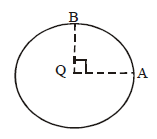Solution: A circle around a point charge represents an equipotential surface (V= Kq/r throughout the perimeter), and work done on an equipotential surface is zero.
QUESTION: 3

### Two spheres having radii of 5 cm and 10 cm bear identical charge of 6.6 nC. Find the potential of spheres after they have been connected by a conductor, assume that the spheres are at large distance from each other

Solution:
QUESTION: 4

An ideal electrical cell does 5 joule of work in carrying 10 coulomb of charge around a closed circuit. The heat loss or energy consumption in the circuit outside the battery is

Solution:
QUESTION: 5

A charged particle of mass 0.1 g is in equilibrium in an electric field E = 98 N/C. The charge on the particle is

Solution:
QUESTION: 6

An electric charge ‘q’ is lying at the open end of a cylindrical vessel. The flux coming out of the cylinder will be

Solution: If you put a another cylinder on it then charge will completely in side and flux will distribute half half so flux =q/2εₒ
QUESTION: 7

On moving a charge of 20 coulombs by 2cm, 2J of work is done, then the potential difference between the points is

Solution: Va - Vb =W/q.
W= work done :2Jq= charge : 20C Va - Vb = 2/20 = 0.1
QUESTION: 8

The capacitance of a parallel plate condenser does not depend upon

Solution:
QUESTION: 9

A semi-circular are of radius ‘a’ is charged uniformly and the charge per unit length is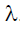. The electric field at its centre is

Solution:
QUESTION: 10

Which of the following cannot be the units of electric intensity?

Solution:
QUESTION: 11

The unit of permittivity of free space ∈0  is

Solution:

Solution :- By coulomb's law the electrostatic force

F = 1/4πε0(q1q2/r2)

⇒ε0 = 1/4πF(q1q2/r2)

Substituting the unit for q, r,and F , we abtain unit of

ε0 = coulomb×coulomb newton−(metre)2

= (coulumb)2 newton −(meter)2

=C2N−m2

QUESTION: 12

Ampere hour is a unit of

Solution:
QUESTION: 13

Six resistors each of value 10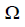, are connected to a 5 V battery as shown in the figure. The reading of ammeter'A' is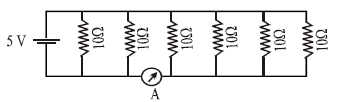Solution:
QUESTION: 14

The potential difference between points ‘A’ and ‘B(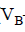-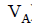)' in the figure if R=0.7is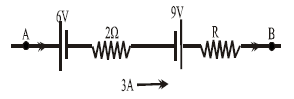Solution: Ans) Applying Kirchhoff's first law
Va - 6 - 2(3) + 9 - (0.7)(3) =Vb
Vb - Va = 9- 6 - 6 - 2.1
=- 3 - 2.1
= - 5.1
QUESTION: 15

If 2% of the main current is to be passed through the galvanometer of resistance G. the resistance of shunt required is

Solution:
Let total current through the circuit be I.
Then, 0.02I current is to be passed through galvanometer of resistance G.
Let the resistance of shunt is Rs .
∵ Shunt are in parallel combination with Galvanometer.
∴ 0.02I * G = (1 - 0.02)I * Rs
⇒0.02G = 0.98 * Rs
⇒Rs = 0.02G/0.98 = G/49

QUESTION: 16

The thermo e.m.f. of a thermocouple is given by e = 2164 t - 6.2 t2 . The neutral temperature and a temperature of inversion are

Solution:
QUESTION: 17

The relation between Faraday constant F, electron charge e, and Avogadro's Number N is

Solution:
QUESTION: 18

In the circuit as shown in Fig. The heat produced by 6resistance due to current flowing in it is 60 calories per second. The heat generated across 3 ohm resistance per second will beSolution:
QUESTION: 19

A thin bar magnet of length 2l and breadth 2b, pole strength p and magnetic moment M is divided into four equal parts with length and breadth of each part being half of the original magnet. Then the pole strength of each part is

Solution:
QUESTION: 20

Susceptibility is positive and small for a ........substance:

Solution:
QUESTION: 21

A magnetic needle lying parallel to a magnetic field requires W units of work to turn it through 600. The torque needed to maintain the needle in this position will be

Solution:
QUESTION: 22

A rectangular coil of 20 turns and area of cross-section 25 sq. cm has a resistance of 100Ω. If a magnetic field which is perpendicular to the plane of coil changes at a rate of 1000 tesla per second, the current in the coil is

Solution:
QUESTION: 23

A bar magnet of magnetic moment μ is bent to form a semi circle. Its magnetic moment will become

Solution:
QUESTION: 24

At a given place on the earth’s surface the horizontal component of magnetic field is 3.2 x 10-5  tesla and the resultant magnetic field is6.4 x 10-5 . The angle of dip at that place is:

Solution:
QUESTION: 25

The mutual inductance of a pair of coils depends on

Solution:
QUESTION: 26

If two bulbs of wattages 25 and 100 respectively, each rated 220V are connected in series with the supply of 440V, which bulb will fuse first?

Solution:
25 Watt bulb will fuse first.

The resistance of each bulb can be calculated from power ratings and voltage ratings.

Power = V*I = V(*V/R) =V^2/R. Therefore:
R = V^/Power.

Resistance of 25 Watt bulb:
R25 = 220*220/25 = =1936 Ohms

Resistance of 100 Watt bulb:
R100 = 220*220/100 = =484 Ohms

When they are connected in series with a supply of 440 V, resultant current will be:
440 V/ (1936 +484) Ohms = 0.1819 Amperes.

The voltage drop across 25 W bulb will be:
V25 =Current * R25
= 0.1818*1936 = 352 Volts. Because of the excessive voltage (much higher than the rated voltage of 220 V), the bulb will fuse in a short time, in less than 1-2 seconds.

Voltage across 100 Watt bulb can be calculated By subtracting 352 from 440 V, which comes to be 88 Volts. To cross check, let us calculate it by multiplication of current and resistance:

Voltage drop across 100 W bulb will be”
V100 = Current * R100
= 0.1818*484 = 88 Volts.

100 Watt bulb will not fuse.
QUESTION: 27

An electron moving in a circular orbit of radius R with a period T is equivalent to a magnetic dipole of dipole moment

Solution: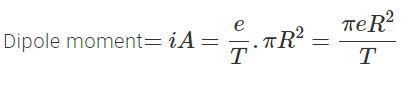QUESTION: 28

Tesla is unit of magnetic

Solution:
QUESTION: 29

A copper rod moves parallel to the horizontal direction. The induced emf developed across its ends due to earth's magnetic field will be maximum at the

Solution:
QUESTION: 30

Figure below shows a resistor and a capacitor connected in series with a 25 V rms alternation supply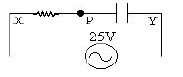What is the r.m.s. potential difference between P and Y given that the r.m.s. potential difference between X and P is 15 V ?

Solution: